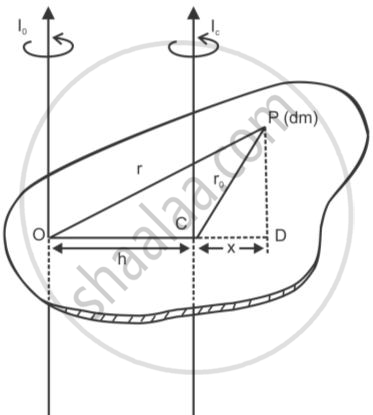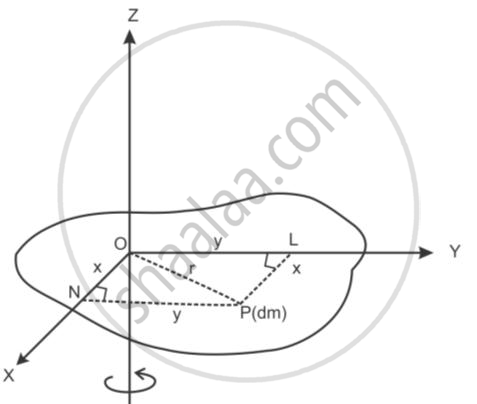HSC Science (General) 12th Board ExamMaharashtra State Board
Share

Prove the Theorem of Parallel Axes About Moment of Inertia - HSC Science (General) 12th Board Exam - Physics

ConceptTheorems of Perpendicular and Parallel Axes

Question

Prove the theorem of parallel axes about moment of inertia

SolutionConsider a rigid of mass ‘M’ rotating about an axis passing through a point ‘O’ and perpendicular to the plane of the figure.Let ‘Io’ be the moment of inertia of the body about an axis passing through point ‘O’.
Take another parallel axis of rotation passing through the centre of mass of the body.
Let ‘Ic’ be the moment of inertia of the body about point ‘C’.
Let the distance between the two parallel axes be OC = h.
OP = r and CP = ro
Take a small element of body of mass ‘dm’ situated at a point P. Join OP and CP, then

I_@=intOP^2dm=intr^2dm

I_C=intCP^2dm=intr_0^2dm

From point P draw a perpendicular to OCproduced.
Let CD = x
From the figure,

OP2 = OD+ PD2

∴OP2=(h + CD)2 + PD2

=h2 + CD2 + 2hCD + PD2

∴OP2= CP2 + h2 + 2hCD ............(CD2 + PD2=CP2)

∴r^2=r_0^2+h^2+2hx

Multiplying the above equation with 'dm' on both the sides and integrating, we get

intr^2dm=intr_0^2dm+inth^2dm+int2hxdm

∴intr^2dm=intr_0^2+inth^2dm+2hintxdm

intxdm=0 as 'C' is the centre of mass and algebriac sum of moments of  all the particles about the center of mass always zero, for body in equilibrium.

therefore intr^2dm=intr_0^2dm+h^2intdm+0.........(Equ.1)

the particles about the centre of mass is always zero, for body in equilibrium.

Butintdm= "mass of the body"

intr^2dm=I_@and intr_0^2dm=I_c

Substituing in equation 1, we get

I0=IC + Mh2
This proves the theorm of parallel axes about moment of inertia.

Is there an error in this question or solution?

APPEARS IN

2015-2016 (March) (with solutions)
Question 4.2.2 | 2.00 marks
2013-2014 (March) (with solutions)
Question 3.1.1 | 4.00 marks
Solution Prove the Theorem of Parallel Axes About Moment of Inertia Concept: Theorems of Perpendicular and Parallel Axes.
S